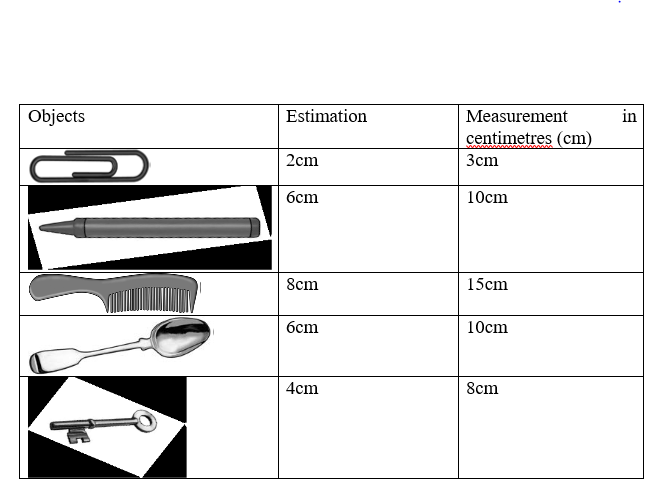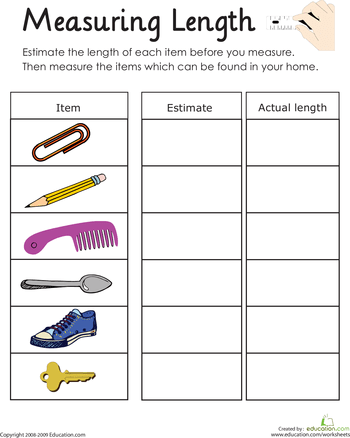# Measurement of Length

Welcome to class.

Length is the distance between two points. Every object in the classroom has different lengths. For example, the classroom is longer than chalkboard; the chalkboard is longer than the desk, and the desk is longer than a notebook. The process is taking the value/ magnitude of length of an object or two points is referred to a measurement.

When an object has more length than the other, then the latter is said to be longer.Length is distance in the horizontals direction while height is distance in vertical direction

IDENTIFYING MEASURING INSTRUMENTS

The instrument of objects can be measured using different measuring instruments such as metre rule, tape rule, foot rule, tape measure, etc

Ruler: is used for measuring and ruling lines.

Measuring tape: is used for different things such as measuring the size of a person when sewing clothes, or measuring something when building. Measuring tapes can bend to measure around different shapes.

Tape measure: is used when building. It is very long and goes back into the

holder when you have finished measuring. If you need a plank of wood that is two metres and 35 cm long, the builders tape can measure the length.

Metre stick is used in the classroom for Mathematics and for ruling lines on the board.

Trundle wheel is an easy way of finding the rough distance from one place to another. They use the trundle wheel at school for marking the fields for sport activities.

Foot rule: is for measuring the size of a foot.

These instruments mentioned above can be used to measure the length, breadth and the height of objects. The S.I unit of length is meter (m) and the metric units are millimeter, centimeter and kilometer.

METRIC UNITS OF LENGTH

The basic unit of length of Meter and the metric units, such as millimetre, centimetre and kilometre, are formed by adding prefix to the basic unit. The prefix (centi, mili, kilo) tells how large or small the measurement is and the base unit (metre) tells the quantity being measured. For example, in centimetre and kilometre, metre is the base unit, and centi and kilo are the prefixes. 1 kilometre is 1000 times larger than 1 metre and 100 centimeter is 1 metre. Therefore, kilometre is larger than centimeter. It should be noted that the units can be converted from one value to another, such as below:

10 millimeters (mm) = 1 centimeter (cm)

10centimeters (cm) = 1 decimeter (dm)

10 decimeters (dm) =1 meter (m)

100 centimeters (cm) = 1 meter (m)

1000 meters (m) = 1 kilometer (km)

when we measure length using the metric system, we know that 10 mm is the same as 1 cm; 100 cm is the same as 1 m and 1 000 m is the same as 1 km.

Short length is measured in centimeter and meter while long distance is measured in Kilometer.

Estimating and measuring

Pupils must learn how to estimate. They should be able to “judge” the length of an object. Sometimes we do not have rulers with us and we have to think about whether a particular object is the right size, so being good at estimating helps.

For pupils who can estimate well, they should get them to use pieces of paper with different sizes with the measurements written on the paper, i.e. a two cm piece of paper, and they can try to estimate with the paper near the object. With a great deal of practice they will get it.

Identifying measuring objectsEvaluation

1. What is length?
2. List any four instrument that can be used to measure a distance
3. The S.I unit of length is ———– (a) metre (b) yard (c) second

ACTIVITYWe have come to the end of the class. If you have any question ask using the comment box. see you in the next class.

Everybody SCREAM💃 ClassNotes evolving to Afrilearn! Get ready for a world-class learning experience🎊

### 2 thoughts on “Measurement of Length”

1.Thank you very much. May the Almighty God bless you and make life easy for you just as you have made things easier for many of us on this platform
I noticed few mistakes.

1.Amen!
Thanks for choosing Classnotes.ng!
Please feel free to recommend the platform to your family and friends. We can also be reached via [email protected]
Cheers!

Don`t copy text!